×#### Thank you for registering.

One of our academic counsellors will contact you within 1 working day.

Click to Chat

1800-1023-196

+91-120-4616500

CART 0

• 0

MY CART (5)

Use Coupon: CART20 and get 20% off on all online Study Material

ITEM
DETAILS
MRP
DISCOUNT
FINAL PRICE
Total Price: Rs.

There are no items in this cart.
Continue Shopping• Complete Physics Course - Class 11
• OFFERED PRICE: Rs. 2,968
• View Details

Gauss Theorem

Table of Content

Electric Flux

​Gauss Law

Point to remember to solve the problem applying Gauss Law

Related Resources

To understand the Gauss law first we have to define the quantity Electric Flux.

Electric Flux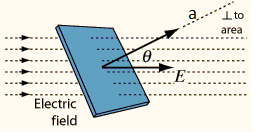The concept of electric ?ux involves a surface and the (vector) values of the electric ?eld at all points of the surface. To introduce the way that ?ux is calculated, we start with a simple case. We will consider a ?at surface of area a and an electric ?eld which is constant (that is, has the same vector value) over the surface.

The surface is characterized by the “area vector” a. This is a vector which points perpendicularly (normal) to the surface and has magnitude a. The surface and its area vector along with the uniform electric ?eld are shown in the side figure.

Actually, there’s a little problem here: There are really two choices for the vector a. (It could have been chosen to point in the opposite direction; it would still be normal to the
surface and have the same magnitude.) However in every problem where we use electric ?ux, it will be made clear which choice is made for the “normal” direction.

In words, electric flux is equal to the number of electric field lines that pass through a particular surface multiplied by the area of the surface. Electric flux is denoted by the symbol ?E.

The electric flux ?E through a Gaussian surface is proportional to the net number of electric field lines passing through that surface.

So,Now, for this simple case, the electric ?ux Φ is given by where theta is the angle between E and a.
?E =Ea cosθ

Here θ is the angle between Electric field vector () and area vector ().

If  is perpendicular to a fl at surface having a total area A, then the electric flux through this surface would be, ?E =Ea. In this case, the flux is just the magnitude of the electric field multiplied by the area of the surface. Flux is a scalar, just an ordinary number; it is not a vector.

Gauss Law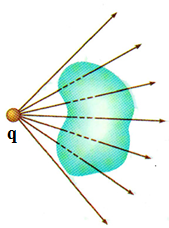The electric flux ?E through any closed surface is equal to the net charge inside the surface, Q inside, divided by ε0:

?E=Qenc/ ε0

Or,

= Qenc/ ε0

So,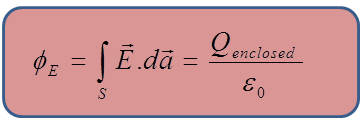Though it’s not obvious, Gauss’s law describes how charges create electric fields. In principle, it can always be used to calculate the electric field of a system of charges or a continuous distribution of charge. In practice, the technique is useful only in a limited number of cases in which there is a high degree of symmetry, such as spheres, cylinders, or planes. With the symmetry of these special shapes, the charges can be surrounded by an imaginary surface, called a Gaussian surface. This imaginary surface is used strictly for mathematical calculation, and need not be an actual, physical surface.

Refer this video for better understanding about Gauss law of electric field

Point to remember to solve the problem applying Gauss Law

(a) Recognize the principle:- To apply Gauss’s Law,you must first calculate the electric flux through a Gaussian surface. The choice of the Gaussian surface is key.

(b) Sketch the problem:- Your drawing should show the charge distribution. Use the symmetry of this distribution to also sketch the electric field. The Gaussian surface should match the symmetry of the electric field.

(c) Identify the relationships:- Your Gaussian surface should satisfy one or more of the following conditions:

(i) has constant magnitude over all or much of the surface and makes a constant angle with the surface. The most convenient surface is one that is perpendicular to  at all (or most) points.

(ii)  may be zero over a portion of the surface. The flux through that part of the surface is zero.

(iii)  may be parallel to some part of the surface. The flux through that part of the surface is zero.

(d) Solve:- First calculate the total electric flux through the entire Gaussian surface. Then fi nd the total electric charge inside the surface and apply Gauss’s law  to solve for  .

Refer this simulation for movement of chargesThe charge Qencl is the net charge enclosed by the arbitrary close surface of our choice.

It does NOT matter where or how much charge is distributed inside the surface.

The charge outside the surface does not contribute.

Electric linked with a surface gives an idea of the number of electric lines of force passing through the surface.

Lines of force leaving a surface produce positive electric flux.

Lines of force entering a surface produce negative electric flux.

Lines of force parallel to the surface do not produce any electric flux.

The integral is over the value of E on a closed surface of our choice in any given situation

Problem 1:-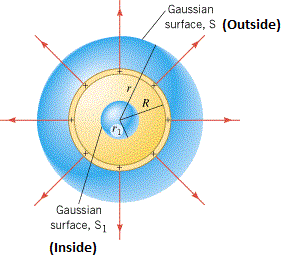Find the field outside a charged solid sphere of radius R and total charge q.

Solution:-

Draw a spherical surface of radius r>R in the below figure. This is called Gaussian surface.

Gauss’s law says that for this surface,

= Qenc/ ε0

Here, Qenc =q

The direction of  is along the radially outward which as the same area vector . So the angle θ between  and  is 0°.

(Since, cos0° = 1)

The magnitude of  is constant over the Gaussian surface, so it comes outside the integral.

= E (4πr2)     (Since, surface area da=4πr2)

As the total charge enclosed by Gaussian surface is q, therefore accordance to Gauss Theorem,

= Qenc/ ε0

E (4πr2) = q/ ε0

So,  = q/4π ε0r2

From the above observation we conclude that, the field outside the sphere is exactly the same as it would have been if all the charge had been concentrated at the center.

_______________________________________________________________________________________________

Problem 2:-An infinite plane carries a uniform surface charge σ. Find its electric field.

Solution:-

To solve this problem, our first job is choose the Gaussian surface. Here the Gaussian surface is “pillbox”. Draw a Gaussian pill box and extends it to equal distances above and below the plane, which as shown in side figure.

In accordance to Gauss law,

= Qenc/ ε0

In this case, Qenc = σA. Here A is the area of the lid of the pillbox. By symmetry, points away from the plane (upward for points above, downward for points below).  So the angle θ between and  is 0° for top and button surfaces and 90° for side surfaces.

Thus, the top and bottom surfaces give,

(Since, θ = 90° )

But the side contributes nothing because the angle θ between  and  is  90° for side surfaces.

Thus, 2EA = Qenc/ ε0 = σA/ ε0

Or,  = (σ/ 2ε0)

Here  is a unit vector pointing away from the surface.

____________________________________________________________________________________________________

Problem 3:-Two infinite parallel planes carry equal but opposite uniform charge densities +σ  and –σ in the side figure. Find the field in each of the three regions: (i) to the left both, (ii) in between  them, (iii) to the right of both.

Solution:-

We observed that, the magnitude of electric field intensity of an infinite plane carries a uniform surface charge σ would be,

E = (σ/ 2ε0)

Using this result, the left plate (positively charged) produces a field (1/2ε0)σ which points away from to the left region (i) and to the right region (ii) and (iii).

The right plate (negatively charged) produces a field (1/2ε0)σ which points towards it (fig:2) to the right regions (i) and (ii) and to the left region (iii).

Thus the electric field intensity in region (i) will be,

E1 = σ/ 2ε0 (-) + σ/ 2ε0()

E1  = 0

Thus the electric field intensity in region (iii) will be,

E3 = σ/ 2ε0 () + σ/ 2ε0(-)

E3  = 0

Thus the electric field intensity in region (ii) will be,

E3 = σ/ 2ε0 () + σ/ 2ε0()

E3  = σ/ ε0 ()

From the above observation, we conclude that, the two fields cancel in regions (i) and (iii). So the field intensity for regions (i) and (iii) is zero. The only region is (ii), where the magnitude of electric field intensity is σ/ ε0 and the direction points to the right, between the plates.Question 1:-

Choose all of the following statements that are true about the electric flux.

(i) The net flux through the whole cubic surface is zero.

(ii) The magnitude of the flux through the top face of the cubic surface is 20 Nm2/C.

(iii) The magnitude of the net flux through the whole cubic surface is  20 Nm2/C.

(a) (i) only    (b) (ii) only     (c) (iii) only    (d) (i) and (ii) only    (e) (ii) and (iii) only

Question 2:-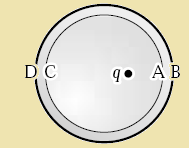A positive point charge q is placed off center inside an uncharged metal sphere insulated from the ground as shown. Where is the induced charge density greatest in magnitude and what is its sign?

(a) A; negative.

(b) A; positive.

(c) B; negative.

(d) B; positive

(e) C; negative

Question 3:-

A point charge +Q is located on the x axis at x = a, and a second point charge –Q is located on the x axis at x = –a. A Gaussian surface with radius r = 2a is centered at the origin.  The flux through this Gaussian surface is,

(a) zero because the negative flux over one hemisphere is equal to the positive flux over the other.

(b) greater than zero.

(c) zero because at every point on the surface the electric field has no component perpendicular to the surface.

(d)  zero because the electric field is zero at every point on the surface.

(e) none of the above

Question 4:-

An astronaut is in an all-metal chamber outside the space station when a solar storm results in the deposit of a large positive charge on the station. Which statement is correct?

(a) The astronaut must abandon the chamber immediately to avoid being electrocuted.

(b) The astronaut will be safe only if she is wearing a spacesuit made of non-conducting materials.

(c) The astronaut does not need to worry: the charge will remain on the outside surface.

(d) The astronaut must abandon the chamber if the electric field on the outside surface becomes greater than the breakdown field of air.

(e)The astronaut must abandon the chamber immediately because the electric field inside the chamber is non-uniform.

Question 5:-

A uniform linear charge density of 4.0 nC/m is distributed along the entire x axis. Consider a spherical (radius = 5.0 cm) surface centered on the origin.  Determine the electric flux through this surface.

(a) 68 N.m2/C      (b) 62  N.m2/C     (c) 45  N.m2/C          (d) 79 N.m2/C           (e) 23 N.m2/C

Question 6:-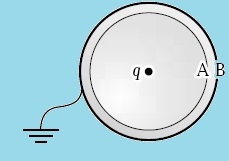A positive point charge q is placed at the center of an uncharged metal sphere insulated from the ground. The outside of the sphere is then grounded as shown. A is the inner surface and B is the outer surface. Which statement is correct?

(a) The charge on A is –q; that on B is +q.

(b) The charge on B is –q; that on A is +q.

(c) The charge is -q/2 on A and on B.

(d) There is no charge on either A or B.

(e) The charge on A is –q; there is no charge on B.Q 1
Q 2
Q 3
Q 4
Q 5
Q 6

d
a
a
c
c
e

Related Resources

You might like to refer Electric Field Intensity.

For getting an idea of the type of questions asked, refer the  Previous Year Question Papers.

To read more, Buy study material of Electrostatics comprising study notes, revision notes, video lectures, previous year solved questions etc. Also browse for more study materials on Physics here.### Course Features

• 101 Video Lectures
• Revision Notes
• Previous Year Papers
• Mind Map
• Study Planner
• NCERT Solutions
• Discussion Forum
• Test paper with Video Solution